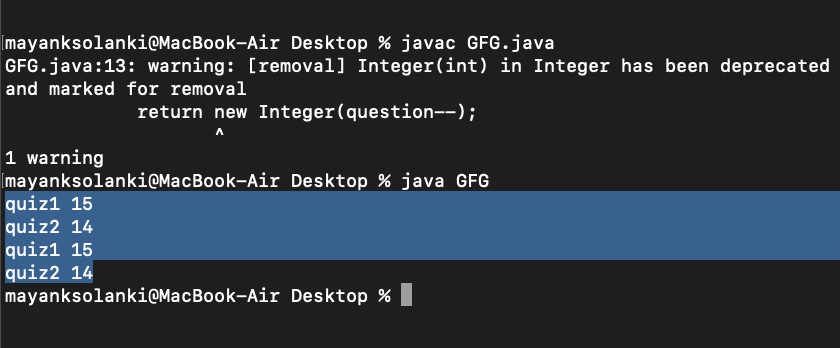# Java.lang.ThreadLocal Class in Java

• Last Updated : 26 May, 2022

This class provides thread-local variables. These variables differ from their normal counterparts in that each thread that accesses one (via its get or set method) has its own, independently initialized copy of the variable. Basically, it is another way to achieve thread safety apart from writing immutable classes. Since the Object is no more shared there is no requirement for Synchronization which can improve the scalability and performance of the application. ThreadLocal provides thread restriction which is an extension of a local variable. ThreadLocal is visible only in a single thread. No two threads can see each other’s thread-local variable. These variables are generally private static fields in classes and maintain their state inside the thread.

Note: ThreadLocal class extends Object class

Constructor: ThreadLocal(): This creates a thread-local variable.

Example 1:

## Java

 `// Java Program to Illustrate ThreadLocal Class``// Via get() and set() Method` `// Class``// ThreadLocalDemo``class` `GFG {` `    ``// Main driver method``    ``public` `static` `void` `main(String[] args)``    ``{` `        ``// Creating objects of ThreadLocal class``        ``ThreadLocal gfg_local``            ``= ``new` `ThreadLocal();` `        ``ThreadLocal gfg = ``new` `ThreadLocal();` `        ``// Now setting custom value``        ``gfg_local.set(``100``);` `        ``// Returns the current thread's value``        ``System.out.println(``"value = "` `+ gfg_local.get());` `        ``// Setting the value``        ``gfg_local.set(``90``);` `        ``// Returns the current thread's value of``        ``System.out.println(``"value = "` `+ gfg_local.get());` `        ``// Setting the value``        ``gfg_local.set(``88.45``);` `        ``// Returns the current thread's value of``        ``System.out.println(``"value = "` `+ gfg_local.get());` `        ``// Setting the value``        ``gfg.set(``"GeeksforGeeks"``);` `        ``// Returning the current thread's value of``        ``System.out.println(``"value = "` `+ gfg.get());``    ``}``}`

Output

```value = 100
value = 90
value = 88.45
value = GeeksforGeeks```

Example 2:

## Java

 `// Java Program to Illustrate ThreadLocal Class``// Via Illustrating remove() Method` `// Class``// ThreadLocalDemo``public` `class` `GFG {` `    ``// Main driver method``    ``public` `static` `void` `main(String[] args)``    ``{``        ``// Creating objects of ThreadLocal class``        ``ThreadLocal gfg_local``            ``= ``new` `ThreadLocal();``        ``ThreadLocal gfg = ``new` `ThreadLocal();` `        ``// Setting the value``        ``gfg_local.set(``100``);` `        ``// Returning the current thread's value``        ``System.out.println(``"value = "` `+ gfg_local.get());` `        ``// Setting the value``        ``gfg_local.set(``90``);` `        ``// Returns the current thread's value of``        ``System.out.println(``"value = "` `+ gfg_local.get());` `        ``// Setting the value``        ``gfg_local.set(``88.45``);` `        ``// Returning the current thread's value of``        ``System.out.println(``"value = "` `+ gfg_local.get());` `        ``// Setting the value``        ``gfg.set(``"GeeksforGeeks"``);` `        ``// Returning the current thread's value of``        ``System.out.println(``"value = "` `+ gfg.get());` `        ``// Removing value using remove() method``        ``gfg.remove();` `        ``// Returning the current thread's value of``        ``System.out.println(``"value = "` `+ gfg.get());` `        ``// Removing vale``        ``gfg_local.remove();` `        ``// Returns the current thread's value of``        ``System.out.println(``"value = "` `+ gfg_local.get());``    ``}``}`

Output

```value = 100
value = 90
value = 88.45
value = GeeksforGeeks
value = null
value = null```

Example 3:

## Java

 `// Java Program to Illustrate ThreadLocal Class``// Via initialValue() Method` `// Importing required classes``import` `java.lang.*;` `// Class 1``// Helper class extending Thread class``class` `NewThread ``extends` `Thread {` `    ``private` `static` `ThreadLocal gfg = ``new` `ThreadLocal() {``        ``protected` `Object initialValue()``        ``{``            ``return` `new` `Integer(question--);``        ``}``    ``};` `    ``private` `static` `int` `question = ``15``;``    ``NewThread(String name)``    ``{``        ``// super keyword refers to parent class instance``        ``super``(name);``        ``start();``    ``}` `    ``// Method``    ``// run() method for Thread``    ``public` `void` `run()``    ``{` `        ``for` `(``int` `i = ``0``; i < ``2``; i++)``            ``System.out.println(getName() + ``" "` `+ gfg.get());``    ``}``}` `// Class 2``// Main class``// ThreadLocalDemo``public` `class` `GFG {` `    ``// Main driver method``    ``public` `static` `void` `main(String[] args)``    ``{` `        ``// Creating threads inside main() method``        ``NewThread t1 = ``new` `NewThread(``"quiz1"``);``        ``NewThread t2 = ``new` `NewThread(``"quiz2"``);``    ``}``}`

Output:This article is contributed by Abhishek Verma. If you like GeeksforGeeks and would like to contribute, you can also write an article using write.geeksforgeeks.org or mail your article to review-team@geeksforgeeks.org. See your article appearing on the GeeksforGeeks main page and help other Geeks. Please write comments if you find anything incorrect, or you want to share more information about the topic discussed above.

My Personal Notes arrow_drop_up